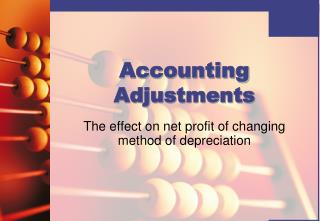DownloadDownload PresentationTélécharger la présentation- - - - - - - - - - - - - - - - - - - - - - - - - - - E N D - - - - - - - - - - - - - - - - - - - - - - - - - - -
##### Presentation Transcript

1. Accounting Adjustments The effect on net profit of changing method of depreciation

2. A business may wish to change the method used to calculate depreciation. This is acceptable provided that they have a good reason for changing the method. Changing method of depreciation

3. For example the method used may no longer be appropriate for the type of asset. If they wish to do this they have to show how this change in method has affected the net profit of the business. Changing method of depreciation

4. Questions will often provide the net profit using the original method of depreciation. You will then be required to calculate the revised net profit using the new method. Changing method of depreciation

5. Gary Kent commenced business on 1 January 2005. The following net profits were reported for the first two years of business: £ 2005 65,000 2006 72,000 Fixed asset transactions during this period were: Machinery £44,000 bought on 1 January 2005 £60,000 bought on 1 July 2006 There were no disposals Depreciation policy in 2005 and 2006 was: 15% on cost straight line method, with rates being applied for each proportion of the year the asset is owned. Early in 2007 consideration was given to changing to the reducing balance method of depreciation on machinery. A rate of 20% would be applied, with the rate being charged for each proportion of the year the machinery is owned. Example

6. a) Calculate the depreciation for machinery in 2005 and 2006, using the original method and rate selected by Gary Kent. b) Calculate the depreciation for machinery in 2005 and 2006, using the alternative method of depreciation. c) Prepare a statement to show the net profit which would have been reported in 2005 and 2006 if the reducing balance method had been used. Required

7. 2005 Machinery cost £44,000 on 1 January 2005 Depreciation 15% straight line method Depreciation = £44,000 x 15% = £6,600 First calculate the depreciation using the original method:

8. 2006 Machine 1 £44,000 x 15% = £6,600 Machine 2 £60,000 x 15% x 6/12 = £4,500 Total depreciation = £6,600 + £4,500 = £11,100

9. 2005 Machinery cost £44,000 on 1 January 2005 Depreciation 20% reducing balance method Depreciation = £44,000 x 20% = £8,800 Next calculate the depreciation using the new method:

10. 2006 Machine 1 Net book value of machinery £44,000 – £8,800 = £35,200 Depreciation = £35,200 x 20% = £7,040 Machine 2 £60,000 x 20% x 6/12 = £6,000 Total depreciation = £7,040 + £6,000 = £13,040

11. Add back the depreciation using the original method. Deduct the depreciation using the new method. We have now calculated the net profit using the new method of depreciation. Method

12. 2005 £ Original net profit 65,000 Add back old method 6,600 71,600 Deduct new method 8,800 Revised net profit 62,800

13. 2006 £ Original net profit 72,000 Add back old method 11,100 83,100 Deduct new method 13,040 Revised net profit 70,060

14. Calculate depreciation using the original method. Calculate depreciation using the new method. Add back the depreciation using the old method to the net profit, deduct the depreciation using the new method. We have now calculated the revised net profit. Summary